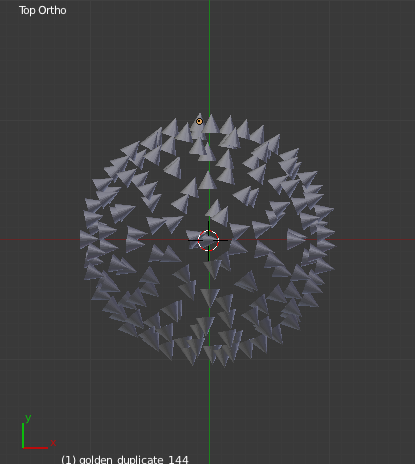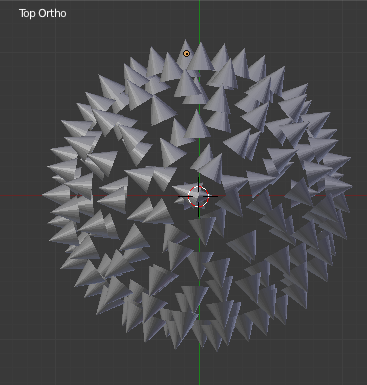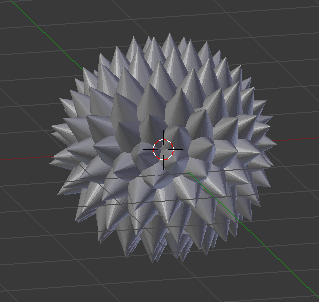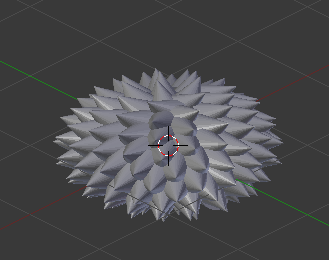# Point Toward Center

I’m duplicating objects over the vertices of a sphere via python.

I’m trying to get them all to point toward the center. For instance, using cones you’d make a spiky ball.

My first way of doing this was to use constraints. It worked great -

But now I’m trying to do it mathematically. Here is my code from the rotation snippet -

`````` obj_center= bpy.data.objects['Golden Points Object'].location

x1, y1, z1 = obj_center, obj_center, obj_center

x2, y2, z2 = vertice.co, vertice.co, vertice.co,

hyp =  math.sqrt((x2 - x1)**2 + (y2 - y1)**2)

opp = y2 - y1

sin = opp / hyp

``````

…which gives the result of attached pic.But of course I’d like the left half to match the right. Any ideas?

PS - I know about dupliverts. I know about constraints! I’m just working on my math and I have special reasons for this workflow.

Ideas: you have an object, a cone (as many as vertices of the ball). Think about an axis of the cone: line from center of bottom to the top
V = (top-center) think it as a vector, the direction of the cone.
Now you move so to say the cones center bottom to the vertex of the ball where it should stay.
Rests to rotate the cone with bottom center fixed such that the direction vector of the axis of cone is opposite of the vector
W = (ball-vertex - center of ball)
I think that is what you want to accomplish, isn’t it?
What you need is a rotation axis and the correct angle.

Rotation ins space leaving a point fixed is ‘complicated’ if the cone’s bottom center is in world (0,0,0) it is easy.
Therefore move cone to the origin (bottom center = (0,0,0)) determine axis and angle, rotate and move cone to the vertex of the ball.

Now trying to find rotation-axis and angle.:
axis = V.cross(W) I think
angle = V.angle(W) #degrees!
and rotationangle is either angle or pi-angle (depends if it should point inside or ouside, just try it)

After rotation move the object(cone) maybe put first the location to the center of the bottom ?! then the translate will probably be OKThats a pretty cool solution, but I was a little stunted (my fault) trying to work it out.

Here is the solution I got - it rotates around the z axis of the circle, now I just have to make it also adjust for x or y

I’m sure there are better ways to do this, like that strange euler angle thing, - but I am still catching up on my math.

I found the 4th quadrant solution to be a little tacky. If someone can correct my math to something more consistent I’d love to hear it.

``````        #ROTATION CALCULATOR
#make adjustment in function depending on which values are negative

obj_center= bpy.data.objects['Golden Points Object'].location

x1, y1, z1 = obj_center, obj_center, obj_center

x2, y2, z2 = vertice.co, vertice.co, vertice.co,

hyp =  math.sqrt((x2 - x1)**2 + (y2 - y1)**2)

opp = y2 - y1

sin = opp / hyp

if x2 &gt; 0 and y2 &gt; 0:

sin = opp/hyp

elif x2 &lt; 0 and y2 &gt; 0:

elif x2 &lt; 0 and y2 &lt; 0:

#since is in third quadrant of unit circle, must add 180 deg

elif x2 &gt; 0 and y2 &lt; 0:

tan = (opp/hyp) / (adj/hyp) -1

#since is in fourth quadrant of unit circle, must add 360 deg

print('Degrees: ', deg)

#/ROTATION CALCULATOR
``````

### Attachmentscould you align the objects to the face normals of the sphere?
that should get them all in the right direction?

Thats my next phase…seems simple enough but it didn’t work first time around. I also found a way to simplify the elif statements, so I’ll try it after that.

some ideas to your script …
If I see something like 180 * ( math.py/180) you could write

math.pi # coming from 180 * ( math.pi/180)

And the same with (opp/hyp) / (adj/hyp)

And is ‘adj’ and ‘hyp’ never zero?

And

# hyp = math.sqrt((x2 - x1)**2 + (y2 - y1)**2)

opp = y2 - y1

This would be great for designing planets.I believe this is a good idea.

Still working on it. Its kind of a slippery thing to work out right now – I’ll post results afterward.

I hope this helps future generations of random googlers. Please improve and update if needed -

Its not the most desirable outcome, but a nice compromise for where I’m at in my math -

Furthest from z on the object, 0 degrees y rotation (local) is applied - so spikes point up. As z-0 is approached, rotation comes closer to 90 degrees. Since the z-rotation is calculated 1-360 degrees, the cone spins, retaining the first angle.

I figured this is good for a sphere, but what if its squashed. It still looks great for my project.

``````#ROTATION CALCULATOR
#make adjustment in function depending on which values are negative

obj_center= bpy.data.objects['Golden Points Object'].location

x1, y1, z1 = obj_center, obj_center, obj_center

x2, y2, z2 = vertice.co, vertice.co, vertice.co,

hyp =  math.sqrt((x2 - x1)**2 + (y2 - y1)**2)

opp = y2 - y1

sin = opp / hyp

if x2 &gt; 0 and y2 &gt; 0: # Quadrant 1

sin = opp/hyp

z_rot = math.asin(sin)

elif x2 &lt; 0 and y2 &gt; 0: # Quadrant 2

z_rot = math.acos(cos)

elif x2 &lt; 0 and y2 &lt; 0: # Quadrant 3

#since is in third quadrant of unit circle, must add 180 deg

elif x2 &gt; 0 and y2 &lt; 0: # Quadrant 4

#since is in fourth quadrant of unit circle, must add 360 deg

#decide y rotation in degrees

y_rot = math.fabs(1-z2) * 90

y_rot = y_rot*(math.pi/180)

#/ROTATION CALCULATOR

``````

Of course it would be much better to catch up and learn matrices/euler angles. All in time I suppose.

### Attachments